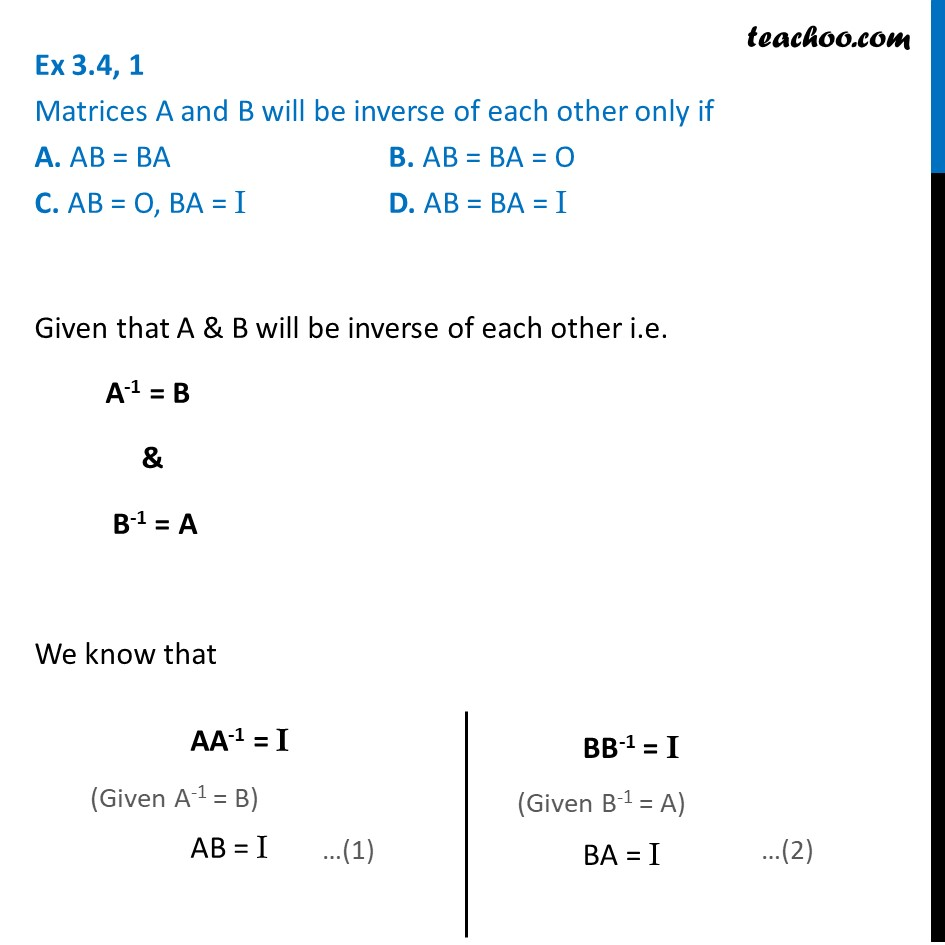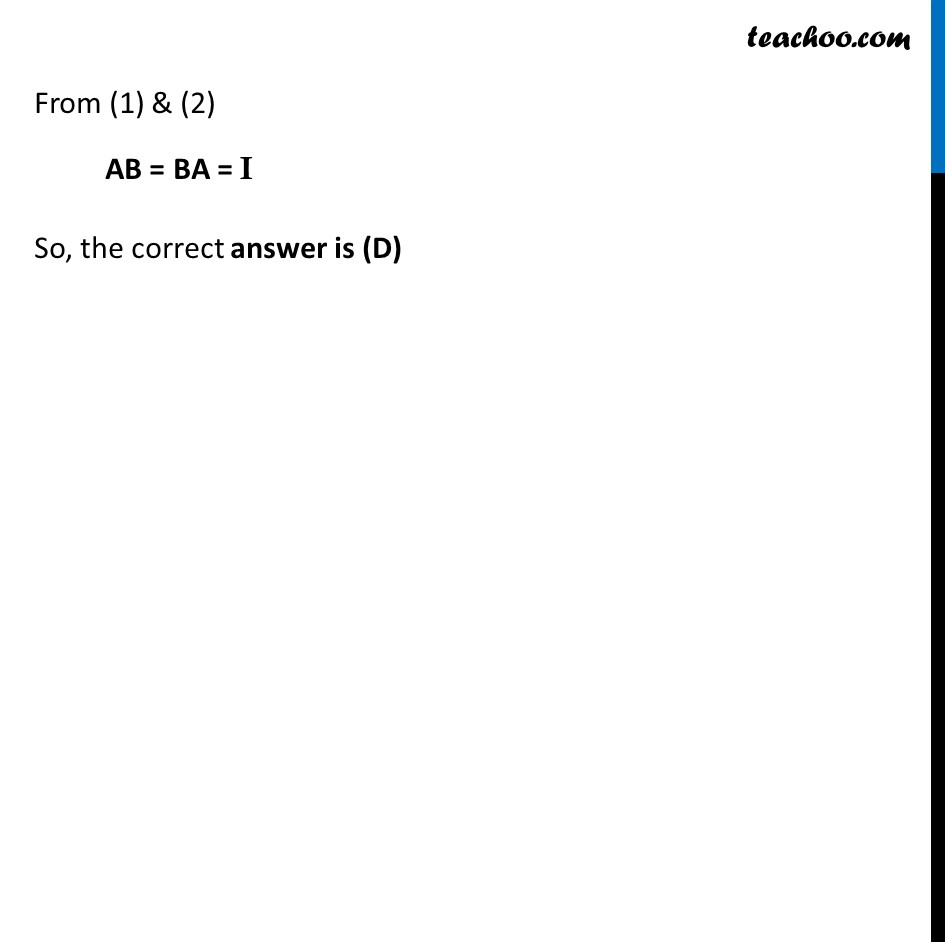Ex 3.4

Chapter 3 Class 12 Matrices
Serial order wiseLearn in your speed, with individual attention - Teachoo Maths 1-on-1 Class

### Transcript

Ex 3.4, 1 Matrices A and B will be inverse of each other only if A. AB = BA B. AB = BA = O C. AB = O, BA = I D. AB = BA = I Given that A & B will be inverse of each other i.e. A-1 = B & B-1 = A We know that From (1) & (2) AB = BA = I So, the correct answer is D From (1) & (2) AB = BA = I So, the correct answer is (D)